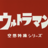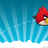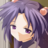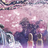V2EX = way to explore
V2EX 是一个关于分享和探索的地方

V2EX  ›  数学

# 求正态分布标准差σ

fuxinya · 242 天前 · 1509 次点击

12 条回复    2021-02-21 21:56:40 +08:001 Ultraman   242 天前2 Ultraman   242 天前1 @Ultraman #1 对不起，，请忽略我😶3 fuxinya   242 天前 @Ultraman 查表也可 只要误差不大就行4 ipwx   241 天前 给楼主一个思路：用 Erf 写出你的方程，然后用牛顿迭代法解。5 ipwx   241 天前这个是要解的方程。在你的问题里，mu=550,l=518,r=560,p=0.86 ipwx   241 天前1 顺便多说一嘴：如果是单边或者对称的就容易多了。因为单边的话，把 erf 函数放到一边，然后直接用 erf 反函数就行了。C 语言和 numpy 都有 erf 和 erf 反函数的数值实现的。。。可惜你是双边不对称7 fuxinya   241 天前 @ipwx 感谢解答, 刚查资料也看见了 erf 反函数 这就去试试8 lcdtyph   241 天前2 能调 mathematica 的话可以直接直接G[x_] := CDF[NormalDistribution[550, sigma], x];FindRoot[G - G == 0.8 , {sigma, 1}]解出来 sigma=11.72339 ipwx   241 天前 @lcdtyph Mathematica 果然牛逼，自动帮搞定各种求根。10 lcdtyph   241 天前1 @ipwx 是的，符号运算太强大了同样功能的代码我用 matlab 写起来这个别扭11 fuxinya   241 天前 @lcdtyph 66612 SharkU   241 天前1 自己写的 Matlab 代码% main scripttolerance = 1e-8;residual = Inf;sigma = 15;h = 1e-6;while residual > tolerance der = (cdfeq(sigma+h) - cdfeq(sigma-h))/h; sigma = sigma -cdfeq(sigma)/der residual = abs(cdfeq(sigma));end% cdfeq functionfunction output = cdfeq( sigma)% 方程: f(sigma) - 0.8 = 0 output = normcdf(560, 550, sigma) - normcdf(518, 550, sigma) - 0.8;end